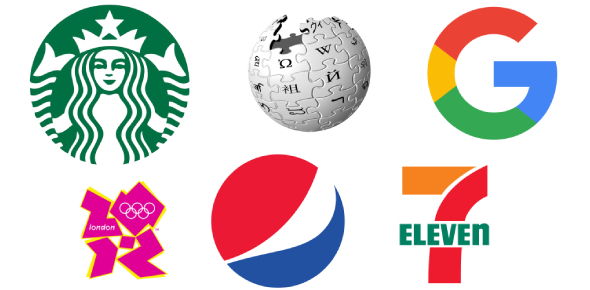# Science Ips/Ibs Final Exam Review

26 Questions | Total Attempts: 124Settings• 1.
What are the base units for the following measurements?a) mass b) volume (liquid) c) length
• A.

A) grams b) liters c) meters

• B.

A) ounces b) fluid ounces c) rulers

• C.

A) kilograms b) liters c) centimeters

• D.

A) lbs b) gallons c) inches

• 2.
What are the steps of the scientific method?
• A.

There is no such thing as a scientific method.

• B.

Conclude, analyze, gather data ,problem, answer

• C.

Problem, hypothesis, experiment, gather data, analyze, conclude

• D.

Problem, experiment, clean-up, hypothesis, analyze, change your hypothesis

• 3.
What changes as a result of the independent variable?
• A.

Dependent variable

• B.

Control group

• C.

Hypothesis

• D.

The problem

• 4.
What is current?
• A.

Opposition of a circuit to the flow of electric current

• B.

The flow of electricity through a conductor

• C.

The voltage

• D.

The same thing as a cation

• 5.
The force of attraction or repulsion is directly proportional to the product of the charges and inversely proportional to the distance between them. This states:
• A.

Coulomb's Law

• B.

Ohm's Law

• C.

The Law of Conservation of Energy

• D.

The third law of thermodynamics

• 6.
What is the equation used to represent Ohm's Law?
• A.

F=ma

• B.

E=mc2

• C.

V=I x R

• D.

2+2=4

• 7.
The definition of amplitude is:
• A.

The maximum displacement of a periodic wave

• B.

The number of occurances within a given period

• C.

The distance between two points in the same phase in consecutive cycles of a wave

• D.

Change in the observed frequency of a wave occuring when the scource whizzes past the observer

• 8.
Light is a a)________ and sound is a b)_________.
• A.

A) longitudinal wave b) transverse wave

• B.

A) transverse wave b) longitudinal wave

• C.

A) solid b) liquid

• D.

A) proton b) electron

• 9.
Refraction is:
• A.

The turning or bending of any wave as it passes from one medium to another

• B.

A disturbance that moves through a medium

• C.

When two waves collide

• D.

The medium a wave travels through

• 10.
Sound travels best through:
• A.

Solids

• B.

Liquids

• C.

Vacuums

• D.

Gases

• 11.
Waves transfer:
• A.

Particles and energy

• B.

Nothing

• C.

Particles

• D.

Energy

• 12.
What do you hear when sound is reflected?
• A.

The doppler effect

• B.

Beats

• C.

Echo

• D.

Cataracts of the eyes

• 13.
Which is NOT a reason for why sunsets are red?
• A.

Blues and purples get scattered at sunset

• B.

Red has the longest wavelength

• C.

The sun is at an angle where it has to travel through the most atmsophere

• D.

Red is a form of ultraviolet radiation

• 14.
What is tempreture?
• A.

The average potential energy

• B.

The average kinetic energy

• C.

The total potential and kinetic energy

• D.

The same thing as thermal energy

• 15.
Burning paper is...
• A.

A chemical reaction

• B.

A physical property

• C.

A physical reaction

• D.

Nuclear fusion

• 16.
Water has a _____ specific heat capacity.
• A.

High

• B.

Low

• C.

Unknown

• D.

Nonexistant

• 17.
Is absolute zero attainable?
• A.

No

• B.

Yes

• C.

Sometimes

• D.

• 18.
Why does a penny become hot when struck by a hammer?
• A.

Energy is transferred from the penny to the hammer

• B.

The hammer is a diffrent metal than the penny

• C.

Energy is transferred from the hammer to the penny

• D.

The penny is able to generate its own heat

• 19.
Heat is measured in:
• A.

Degrees Celsius

• B.

Joules or calories

• C.

Meters

• D.

Newtons

• 20.
Metals are located to the _____ of the periodic table.
• A.

Left

• B.

Right

• C.

There are no metals in the periodic table, trick question

• D.

Top half

• 21.
Noble gases have ______ valence electrons.
• A.

8

• B.

1

• C.

0

• D.

4

• 22.
What type of bond uses prefixes whern being named?
• A.

Metallic

• B.

Ionic

• C.

Covalent

• D.

None

• 23.
How do we balance equations?
• A.

• B.

• C.

• D.

By adding another element to the reactants side

• 24.
Isomers:
• A.

Have the same chemical formula, but different arrangement of atoms

• B.

Have different chemical formulas, but the same arrangement of atoms

• C.

Have nothing in common

• D.

Are identical

• 25.
When an acid and a base react, the products are...
• A.

Acids

• B.

Bases

• C.

Water and a salt

• D.

Usually very dangerous

Related TopicsBack to top